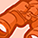# New to Qlik Sense

If you’re new to Qlik Sense, start with this Discussion Board and get up-to-speed quickly.

Announcements
Customer & Partners, DEC. 9, 11 AM ET: Qlik Product & Strategy Roadmap Session: Data Analytics REGISTER NOW
cancel
Showing results for
Did you mean:Contributor III

## Variable in load editor with Set analysis expression

Hi,

I am trying to load variables in the load editor. The variables include set analysis expressions.

Example:

Let vKPI_Budget = SUM({\$<MonthNum ={'>=\$(=vFMonth)<=\$(=vTMonth)'}, Year={"\$(=vYear)"}>}[Budget] );

How can I load this in the Data Load editor? It gives issues with the {, '' and "".

I prefer not doing it via an external excel with all variables, I know that works.

1 Solution

Accepted SolutionsMVP

Try this:

LET vKPI_Budget = 'Sum({\$' & '<MonthNum = {'>=\$' & '(=vFMonth)<=\$' & '(=vTMonth)'}, Year={"\$' & '(=vYear)"}>}[Budget])';

8 RepliesMVP

Try this:

LET vKPI_Budget = 'Sum({\$' & '<MonthNum = {'>=\$' & '(=vFMonth)<=\$' & '(=vTMonth)'}, Year={"\$' & '(=vYear)"}>}[Budget])';MVP

This might even work without the first break, but I am not 100% sure

LET vKPI_Budget = 'Sum({\$<MonthNum = {'>=\$' & '(=vFMonth)<=\$' & '(=vTMonth)'}, Year={"\$' & '(=vYear)"}>}[Budget])';Contributor III
Author

After I replaced the single quotes by the double quotes and used your technique to break it apart... it worked.

Thanks Sunny TPartner

Can you post what your final solution was?Contributor III
Author

Hi Tim,

It's:

Let vKPI_Budget = 'SUM({\$' & '<MonthNum ={">=\$' & '(=vFMonth)<=\$' & '(=vTMonth)"}, Year={"\$' & '(=vYear)"}>}[Budget] )';Creator III

I'm trying to do something similar but I just can't get it to work. Here's my variable statement in my load script:

let vExp = '=Sum({<Period={\$(vPriorPer)},Year={\$(vYr)}>} ExtendedPrice)';

This gets evaluated as a formula, but doesn't evaluate to an actual value. My goal is get the value of the expression into the variable so I can load it to another table inline.

Thanks.MVP

Try this:

SET vExp = '=Sum({<Period={#(vPriorPer)},Year={#(vYr)}>} ExtendedPrice)';

LET vExp2 = Replace(\$(vExp), '#', '\$');Creator III

Thanks for the suggestion, but I don't think it's working. I get an error "unexpected token Sum, expected nothing.", on the vExp2 variable and then it evaluates to Null.Tags
Community Browser